# Classify the following soils using the U.S. Department of Agriculture textural classification chart.### Principles of Geotechnical Enginee...

9th Edition
Braja M. Das + 1 other
Publisher: Cengage Learning
ISBN: 9781305970939### Principles of Geotechnical Enginee...

9th Edition
Braja M. Das + 1 other
Publisher: Cengage Learning
ISBN: 9781305970939

#### Solutions

Chapter
Section
Chapter 5, Problem 5.1P
Textbook Problem

## Classify the following soils using the U.S. Department of Agriculture textural classification chart.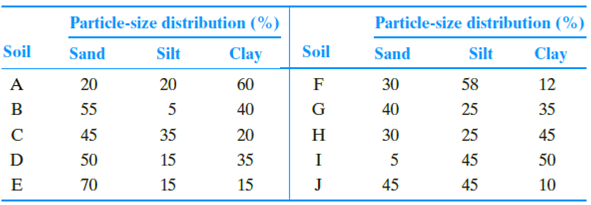Expert Solution
To determine

The classification of soil based on United States department of agriculture textural classification chart.

The classification of soil A as per the United States department of agriculture textural classification chart is Clay_.

The classification of soil B as per the United States department of agriculture textural classification chart is Sandyclay_.

The classification of soil C as per the United States department of agriculture textural classification chart is Loam_.

The classification of soil D as per the United States department of agriculture textural classification chart is Sandyclayandsandyclayloam_.

The classification of soil E as per the United States department of agriculture textural classification chart is Sandyloam_.

The classification of soil F as per the United States department of agriculture textural classification chart is Siltyloam_.

The classification of soil G as per the United States department of agriculture textural classification chart is Clayloam_.

The classification of soil H as per the United States department of agriculture textural classification chart is Clay_.

The classification of soil I as per the United States department of agriculture textural classification chart is Siltyclay_.

The classification of soil J as per the United States department of agriculture textural classification chart is Loam_.

### Explanation of Solution

Calculation:

Consider soil A:

Refer Figure (5.1) “U.S. Department of agriculture textural classification (USDA)”

in the text book.

Mark the percentage of sand for soil A as 20 %, percentage of silt as 20 %, and percentage of clay as 60 % in Figure (5.1) and mark the point of intersection of three lines.

Show the percentage of sand, silt, and clay of soil A as in Figure (1).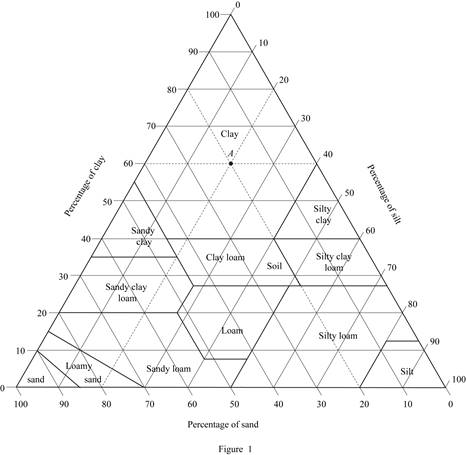Refer Figure (1).

Hence, the classification of soil A as per the United States department of agriculture textural classification chart is Clay_.

Consider soil B:

Mark the percentage of sand for soil B as 55 %, percentage of silt as 5 %, and percentage of clay as 40 % in Figure (5.1) and mark the point of intersection of three lines.

Show the percentage of sand, silt, and clay of soil B as in Figure (2).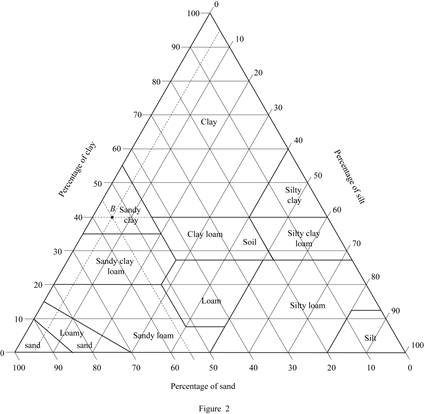Refer Figure (2).

Thus, classification of soil B as per the United States department of agriculture textural classification chart is Sandyclay_.

Consider soil C:

Mark the percentage of sand for soil C as 45 %, percentage of silt as 35 %, and percentage of clay as 20 % in Figure (5.1) and mark the point of intersection of three lines.

Show the percentage of sand, silt, and clay of soil C as in Figure (3).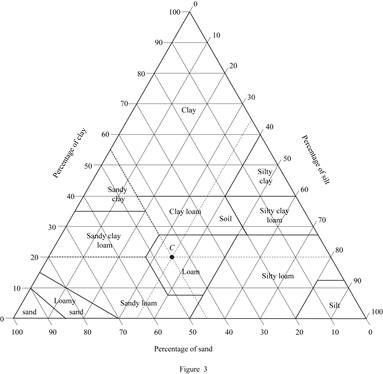Refer Figure (3).

The classification of soil C as per the United States department of agriculture textural classification chart is Loam_.

Consider soil D:

Mark the percentage of sand for soil D as 50 %, percentage of silt as 15 %, and percentage of clay as 35 % in Figure (5.1) and mark the point of intersection of three lines.

Show the percentage of sand, silt, and clay of soil D as in Figure (4).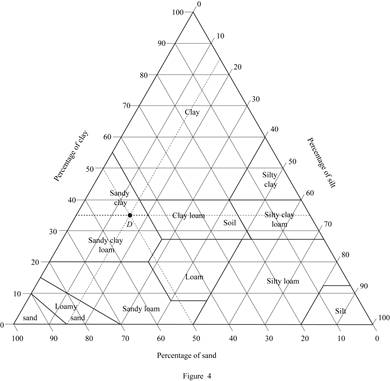Refer Figure (4).

The classification of soil D as per the United States department of agriculture textural classification chart is Sandyclayandsandyclayloam_.

Consider soil E:

Mark the percentage of sand for soil E as 70 %, percentage of silt as 15 %, and percentage of clay as 15 % in Figure (5.1) and mark the point of intersection of three lines.

Show the percentage of sand, silt, and clay of soil E as in Figure (5).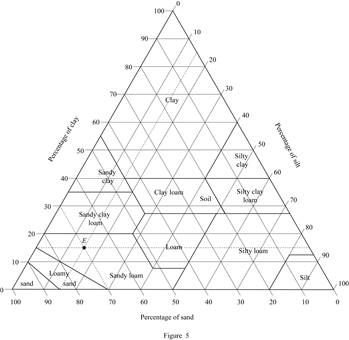Refer Figure (5).

The classification of soil E as per the United States department of agriculture textural classification chart is Sandyloam_.

Consider soil F:

Refer Figure (5.1) “U.S. Department of agriculture textural classification (USDA)”

in the text book.

Mark the percentage of sand for soil F as 30 %, percentage of silt as 58 %, and percentage of clay as 12 % in Figure (5.1) and mark the point of intersection of three lines.

Show the percentage of sand, silt, and clay of soil F as in Figure (6).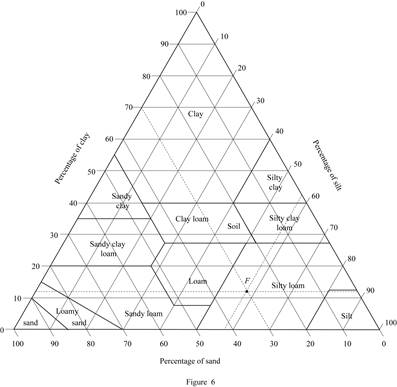Refer Figure (6).

Hence, the classification of soil F as per the United States department of agriculture textural classification chart is Siltyloam_.

Consider soil G:

Mark the percentage of sand for soil G as 40 %, percentage of silt as 25 %, and percentage of clay as 35 % in Figure (5.1) and mark the point of intersection of three lines.

Show the percentage of sand, silt, and clay of soil G as in Figure (7).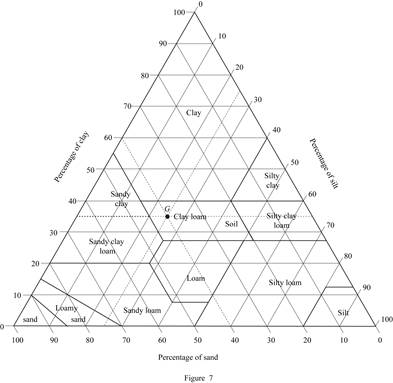Refer Figure (7).

Thus, classification of soil G as per the United States department of agriculture textural classification chart is Clayloam_.

Consider soil H:

Mark the percentage of sand for soil H as 30 %, percentage of silt as 25 %, and percentage of clay as 45 % in Figure (5.1) and mark the point of intersection of three lines.

Show the percentage of sand, silt, and clay of soil H as in Figure (8).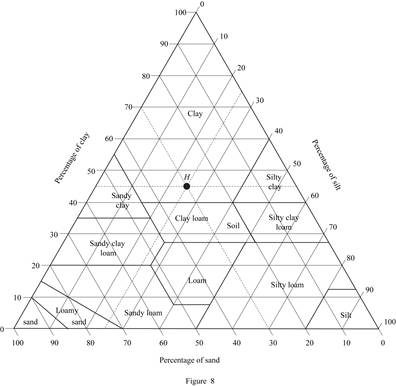Refer Figure (8).

The classification of soil H as per the United States department of agriculture textural classification chart is Clay_.

Consider soil I:

Mark the percentage of sand for soil I as 5 %, percentage of silt as 45 %, and percentage of clay as 50 % in Figure (5.1) and mark the point of intersection of three lines.

Show the percentage of sand, silt, and clay of soil I as in Figure (9).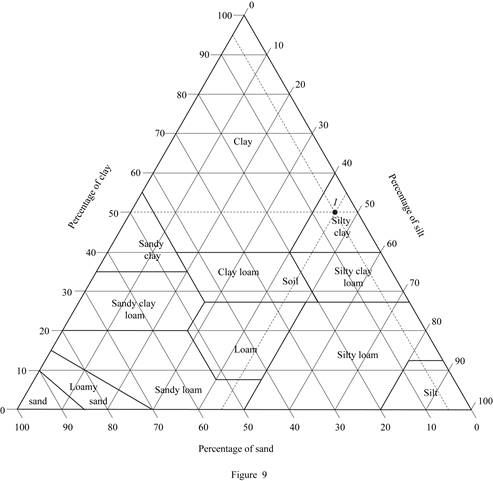Refer Figure (9).

The classification of soil I as per the United States department of agriculture textural classification chart is Siltyclay_.

Consider soil J:

Mark the percentage of sand for soil J as 45 %, percentage of silt as 45 %, and percentage of clay as 10 % in Figure (5.1) and mark the point of intersection of three lines.

Show the percentage of sand, silt, and clay of soil J as in Figure (10).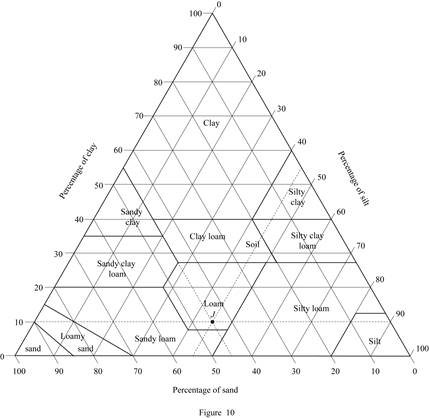Refer Figure (10).

The classification of soil J as per the United States department of agriculture textural classification chart is Loam_.

### Want to see more full solutions like this?

Subscribe now to access step-by-step solutions to millions of textbook problems written by subject matter experts!

Get Solutions

### Want to see more full solutions like this?

Subscribe now to access step-by-step solutions to millions of textbook problems written by subject matter experts!

Get Solutions

Find more solutions based on key concepts
Show solutions
Which layer of the Earth contains the most amount of mass?

Engineering Fundamentals: An Introduction to Engineering (MindTap Course List)

What abrasive is commonly used to grind steels?

Precision Machining Technology (MindTap Course List)

What is a relational diagram? Give an example.

Database Systems: Design, Implementation, & Management

What enterprise resource planning (ERP)? What is supply chain management (SCM)?

Systems Analysis and Design (Shelly Cashman Series) (MindTap Course List)

What is risk management?

Management Of Information Security

Replace the three forces by a single equivalent force R. Express your answer in vector form.

International Edition---engineering Mechanics: Statics, 4th Edition

What can happen if too much power is being carried by a cable?

Welding: Principles and Applications (MindTap Course List)

How do e-commerce and m-commerce differ? (82)

Enhanced Discovering Computers 2017 (Shelly Cashman Series) (MindTap Course List)

Why is a BPDU filter needed at the demarc?

Network+ Guide to Networks (MindTap Course List)

If your motherboard supports ECC DDR3 memory, can you substitute non-ECC DDR3 memory?

A+ Guide to Hardware (Standalone Book) (MindTap Course List)